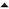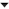## VGA

The verilog provided at 4.3_psp_lcd can also be used to drive a VGA display. Only the timing and resolution in h_sync, v_sync and in_picture and the pixel clock in clk should be changed to the correct values as explained in vga_timing.

#### Example for 640x480

##### in_picture module
Change the values of the front and backporch in in_picture by changing
C: in_picture
 ```1 2 3 ``` ```assign h_pixel = h_addr - 10'h30; assign v_line = v_addr - 9'h10; assign in_picture = (h_addr > 10'h30 && h_addr < 10'h213 && v_addr > 9'h10 && v_addr < 9'h120)?1'b1:1'b0;```

to
C: in_picture
 ```1 2 3 ``` ```assign h_pixel = h_addr - 10'h90; assign v_line = v_addr - 9'h23; assign in_picture = (h_addr > 10'h90 && h_addr < 10'h310 && v_addr > 9'h23 && v_addr < 9'h1E2)?1'b1:1'b0;```
##### clk module
Change the pixel clock in clk by changing
C: clk
 ```1 2 3 4 5 6 7 8 9 ``` ``` always @ (posedge clk_50MHz) begin if (count == 3'h5) count <= 0; else count <= count + 3'h1; end assign clk_9MHz = count;```

to
C: clk
 ```1 2 3 4 ``` ``` always @ (posedge clk_50MHz) count <= ~count; assign clk_9MHz = count;```
##### v_sync module
In v_sync module, change the sync pulse duration by changing the following:
C: v_sync
 ```1 2 3 4 5 6 7 ``` ``` if (count == 286) count <= 9'h0; if (count < 12) sync <= 1'h0; else sync <= 1'h1;```

to
C: v_sync
 ```1 2 3 4 5 6 7 ``` ``` if (count == 525) count <= 9'h0; if (count < 2) sync <= 1'h0; else sync <= 1'h1;```
##### h_sync module
In h_sync module, change the sync pulse duration by changing the following:
C: h_sync
 ```1 2 3 4 5 6 7 ``` ``` if (count == 525) //525 count <= 10'h0; if (count < 45) //45 sync <= 1'h0; else sync <= 1'h1;```

to
C: h_sync
 ```1 2 3 4 5 6 7 ``` ``` if (count == 799) count <= 10'h0; if (count < 96) sync <= 1'h0; else sync <= 1'h1;```
##### Signal widths
Also, the width of h_addr, v_addr, h_pixel, v_line and address should be changed and the depth of the display memory
old new 10 11 9 10 10 11 9 10 10 11 2048 4096

#### Result

This will result in a display memory of 128×32 character with 30×80 visible character at a resolution of 680×480 (60 Hz refresh rate). It will use up 64 kB (70%) of the available ram bits. It is recommended to reduce the font table to 64 characters and (if needed) to increase to size of the characters to 16×32 instead of 8×16. This is done by shifting the h_addr and v_addr lines one to the left and increasing the shift register (in the color module) every other clock cycle.02-'10 4.3" PSP LCD01-'10 IBM font